Subject:
Mathematics
Material Type:
Lesson Plan
Level:
Middle School
7
Provider:
Pearson
Tags:
Language:
English
Media Formats:
Text/HTML

# Gallery Problems Exercise# Gallery Overview

Allow students who have a clear understanding of the content thus far in the unit to work on Gallery problems of their choosing. You can then use this time to provide additional help to students who need review of the unit’s concepts or to assist students who may have fallen behind on work.

# Gallery Descriptions

Temperature Changes
Students solve a puzzle by using clues about the temperatures and temperature changes between several cities.

Time Zones
Students use integers to solve problems about times in different world time zones.

Build Expressions
Pairs of students play a game in which they use cards to build two expressions that are as close in value as possible.

Hexagon Puzzle
Students assemble triangular puzzle pieces by matching the problems and answers on their sides. When the puzzle is complete, the pieces will form a large hexagon.

Equivalent Expressions
Students sort expressions into groups that have the same value.

Are They Equivalent?
Students decide when given expressions will have the same value as ab.

The locations of a and b on a number line are shown, and students must graph −a, −b, ab, b − a, a + b,
and −ab.

p and n
Students decide whether statements about a positive number p and a negative number n are true for all, some, or no values of p and n.

# Temperature Changes

City Temperature Cards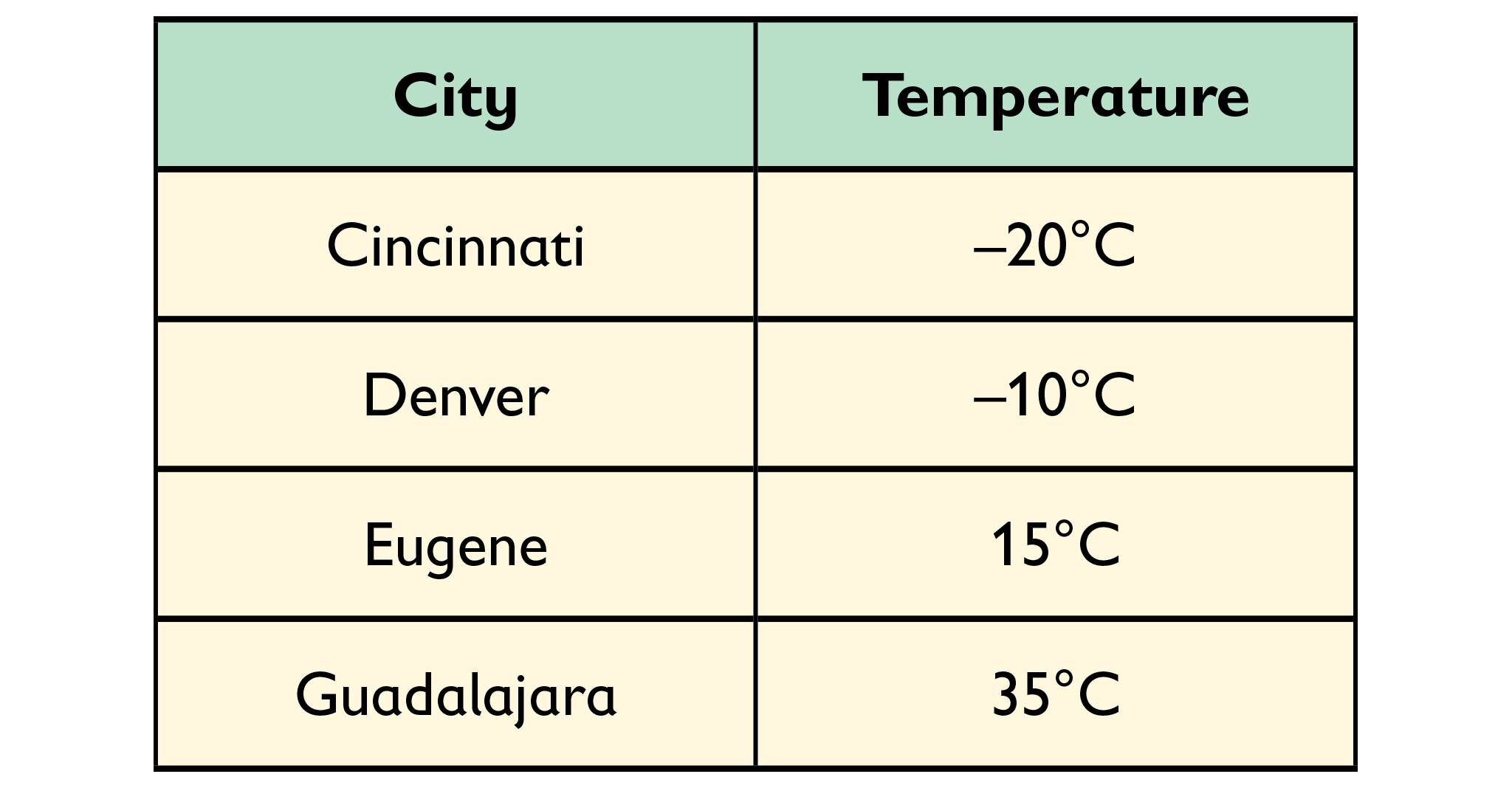Temperature Changes Cards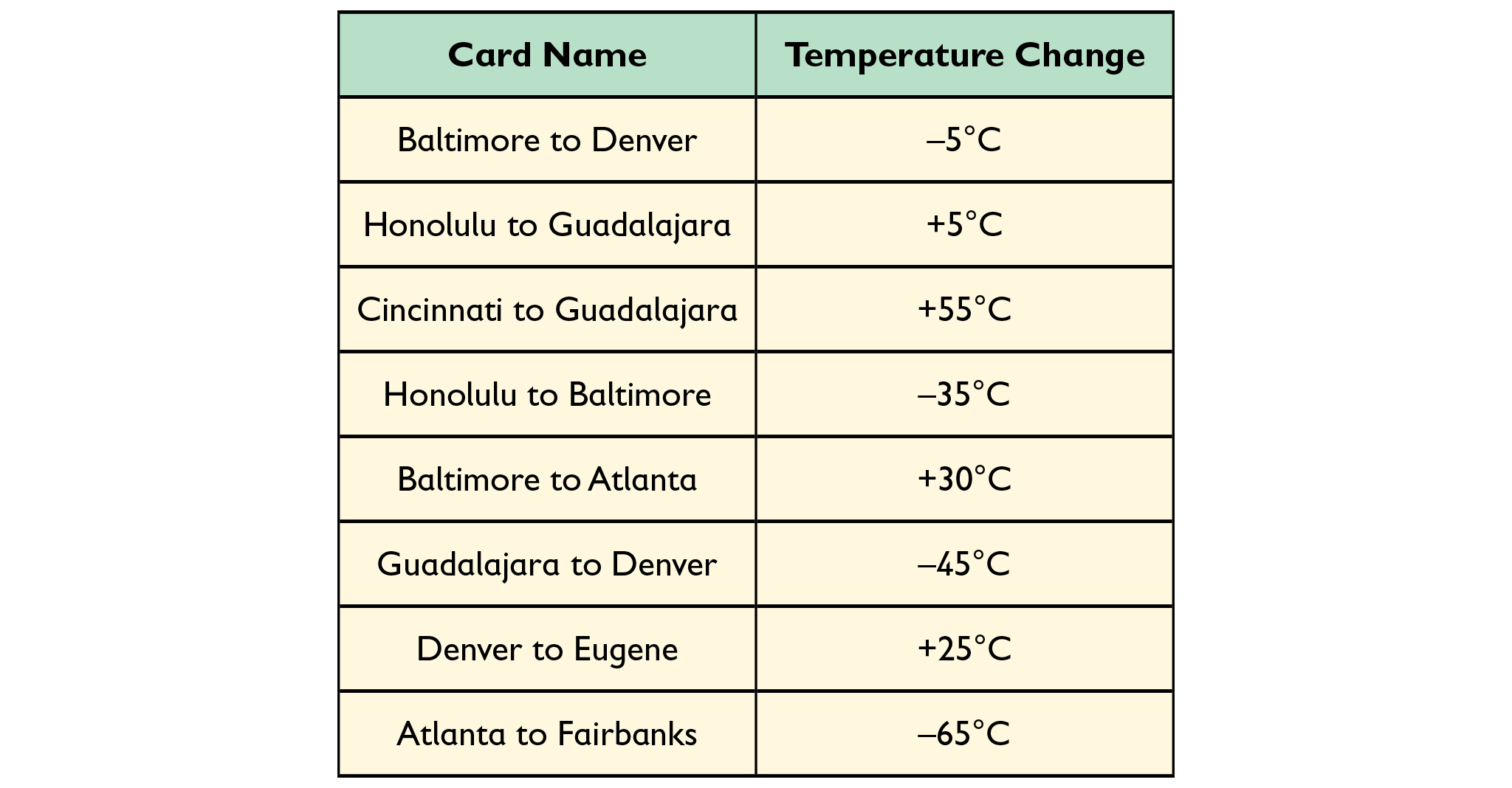# Temperature Changes

In this activity, City Temperature cards show the temperature in eight cities. The Temperature Change cards show the temperature change from one city to another. Many of the cards have missing information that you will need to fill in.

1. Choose any City Temperature card, and then find a Temperature Change card for that city.

2. Find the City Temperature card for the other city on theTemperature Change card.

3. Use the information on the three cards to calculate the missing temperature or temperature change and fill in the value. For example, “Cincinnati to Baltimore +15°C" " means add 15°C to Cincinnati’s temperature to get Baltimore’s temperature.

4. Continue makiong Temperature Change and City Temperature card pairs until you have filled in all the values. Check your work by choosing different combinations of City Temperature and Temperature Change cards.

# Time Zones

1. Timeline: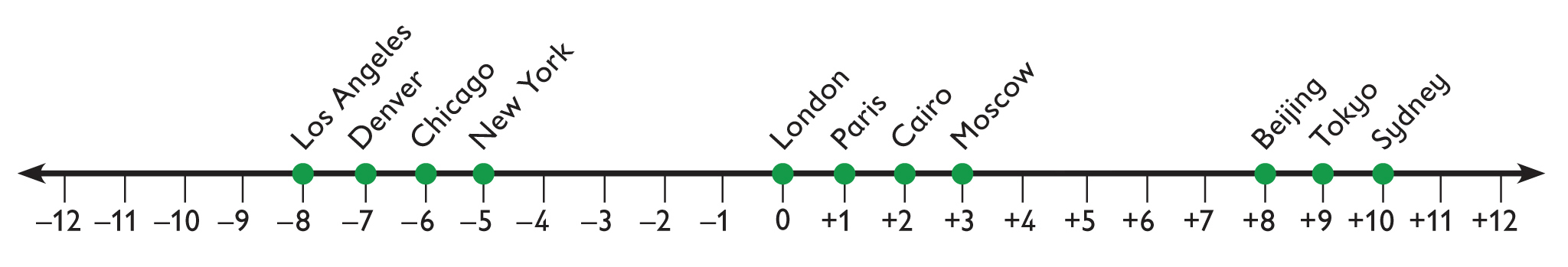2. Time table: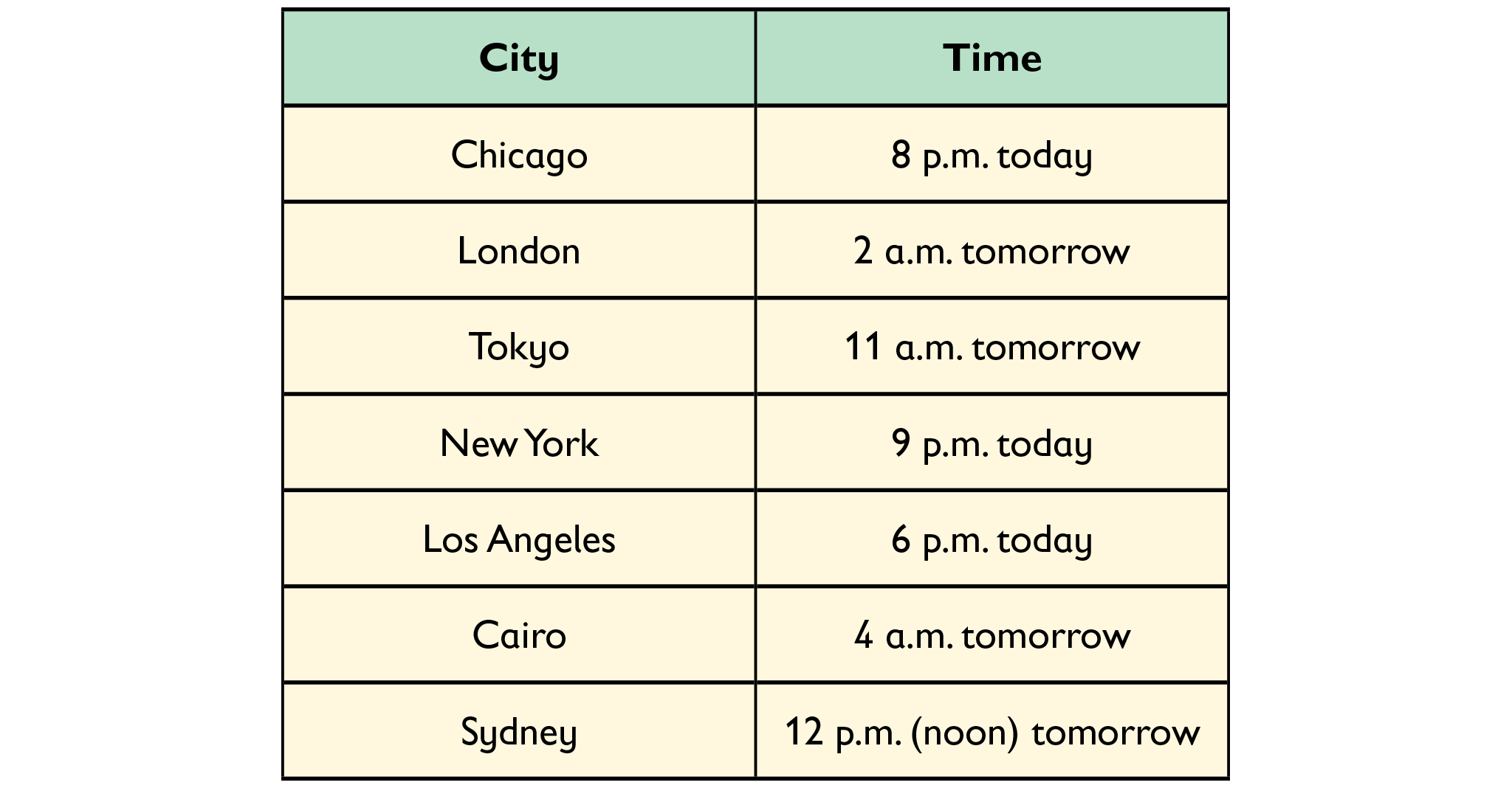3. It is 9 a.m. on Monday in New York.

# Time Zones

Standard Time at different places around the world varies from 12 hours ahead of Greenwich Mean Time (0 hours in England) to 12 hours behind.

1. Locate the point on the number line representing the time zone for each of the cities shown in the map. Use positive numbers for the time zones that are ahead of Greenwich Mean Time and negative numbers for the time zones that are behind.

2. Use the number line from Problem 1 to complete the table.

3. If it is midnight on Tuesday in Sydney, Australia, what time and day is it in New York?

HANDOUT: Cities and Time Zones

# Build Expressions

Create two expressions by combining the numbers and the $+$ and the $-$ operators. Your goal is to obtain two expressions that are as close in value as possible. Each expression can have one, two, or three numbers. You must use all four numbers.

Make teams of two players.

1. Calculate the result of each expression and enter it into the “Result” column of the Scoring Table.
2. Calculate your score for the turn by finding the absolute value of the difference in value of the two expressions.
3. Repeat with three different sets of numbers until the Scoring Table is complete. Each team is made up of two players. Compare your team's total score with a different team's total score. The team with the lowest score wins.

HANDOUT: Building and Comparing Expressions

# Hexagon Puzzle

Completed puzzle: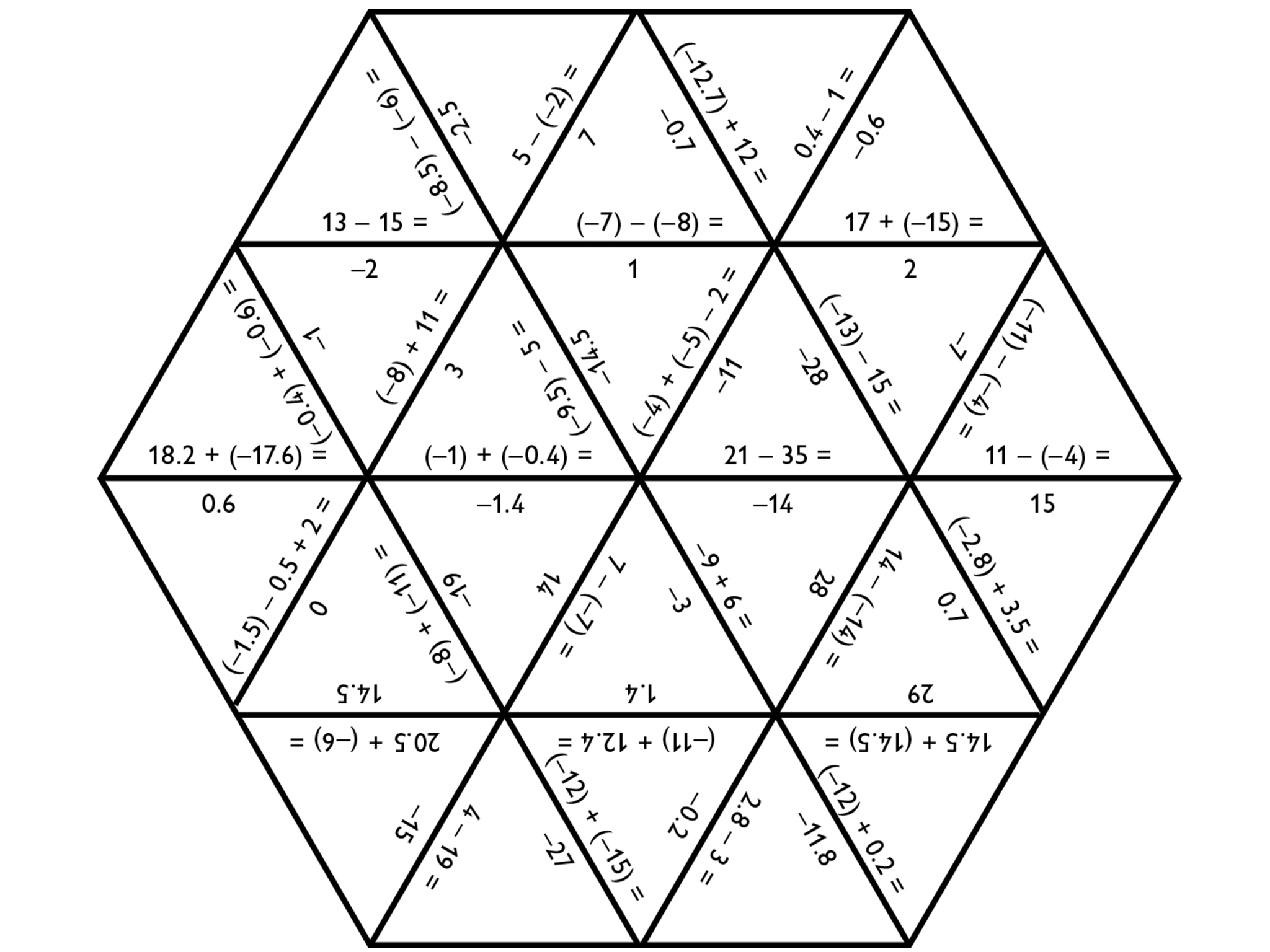# Hexagon Puzzle

Each triangle piece has calculations and answers on its sides. Arrange the triangles so that each side with a calculation is matched to a side with the correct answer. When the triangles are arranged correctly, they will form a large hexagon.

HANDOUT: Hexagon Puzzle

# Equivalent Expressions

The expressions can be sorted into four groups.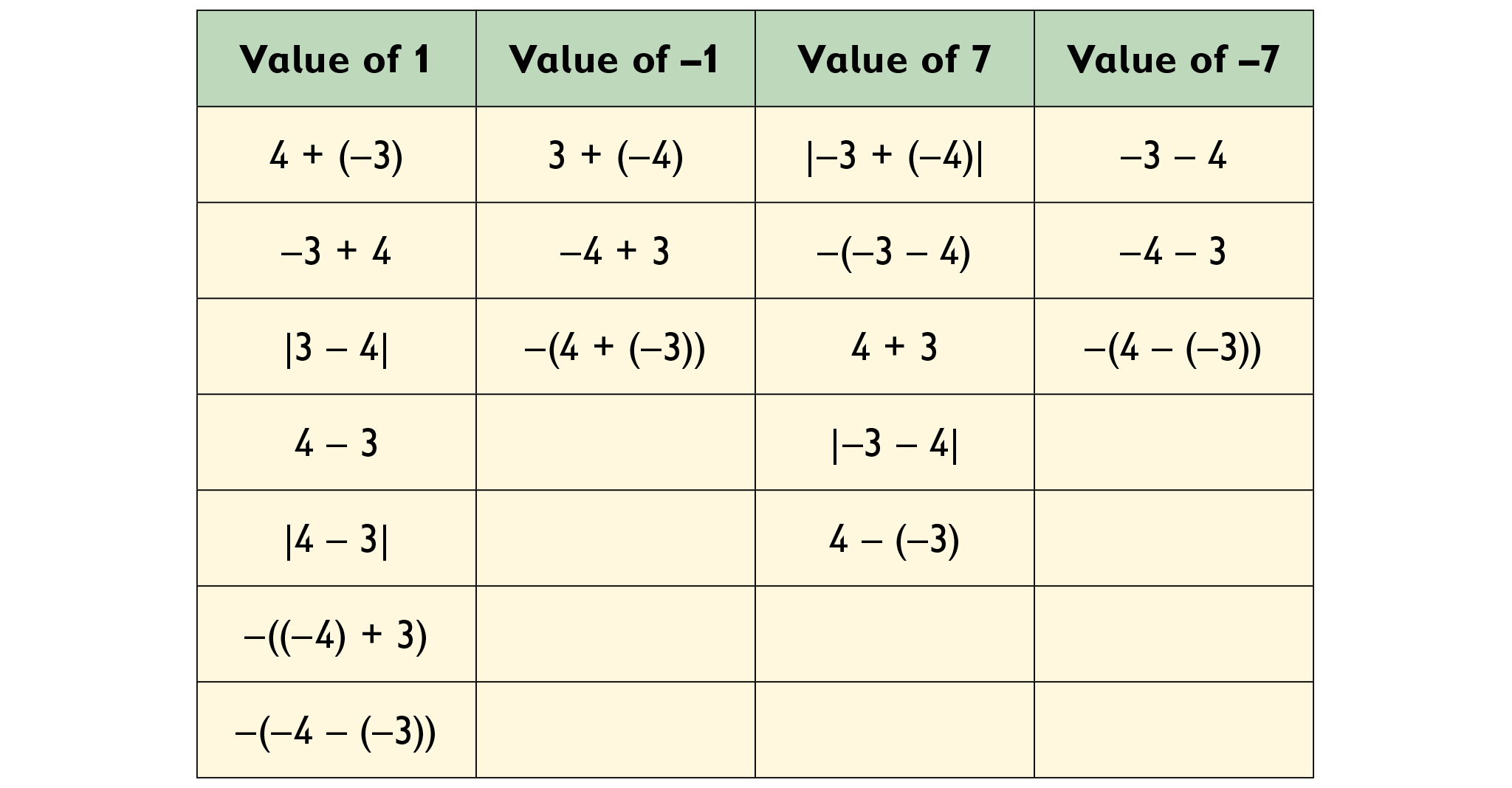# Equivalent Expressions

Sort these expressions into groups with the same value.

HANDOUT: Equivalent Expressions

# Are They Equivalent?

1.
• Not equivalent. For example, when a = 0 and b = 5, ab = −5, but |a b| = 5.
• Equivalent. Using the commutative property, −b + a = a + (−b) = ab.
• Not equivalent. For example, when a = 5 and b = 0, ab = 5, but −(ab) = −5.
• Equivalent. Subtracting b is the same as adding its opposite, so ab = a + (−b).
• Not equivalent. For example, when a = 0 and b = 5, ab = −5, but |ba| = 5.
• Not equivalent. If ab, then ab ≠ 0.
• Equivalent. −(−a) + (−b) = (−1)(−a) − b = ab.

2.    Expression 1: ab will have the same value as |ab| when a is greater than or equal to b.

Expression 3: −(ab) will have the same value as ab only when a = b, in which case both expressions will equal 0. For all other values of a and b, the two expressions will be (non-zero) opposites.

Expression 5: |ba| will have the same value as (ab) whenever a is greater than or equal to b.

Expression 6: (ab) will equal 0 when a = b.

# Are They Equivalent?

Two algebraic expressions are equivalent if they are equal for all variable values.

1. Determine whether each expression is equivalent to ab. If it is, explain how you know. If it is not, give values for a and b for which the expression is not equal to ab.

•  $|a-b|$

•  $-b+a$

•  $-\left(a-b\right)$

•  $a+\left(-b\right)$

•  $|b-a|$

•  $0$

•  $-\left(-a\right)+\left(-b\right)$

2. Consider the expressions in Problem 1 that are not equivalent to ab. Determine whether each expression would have the same value as ab under certain conditions. If so, specify those conditions.

1. Number lines: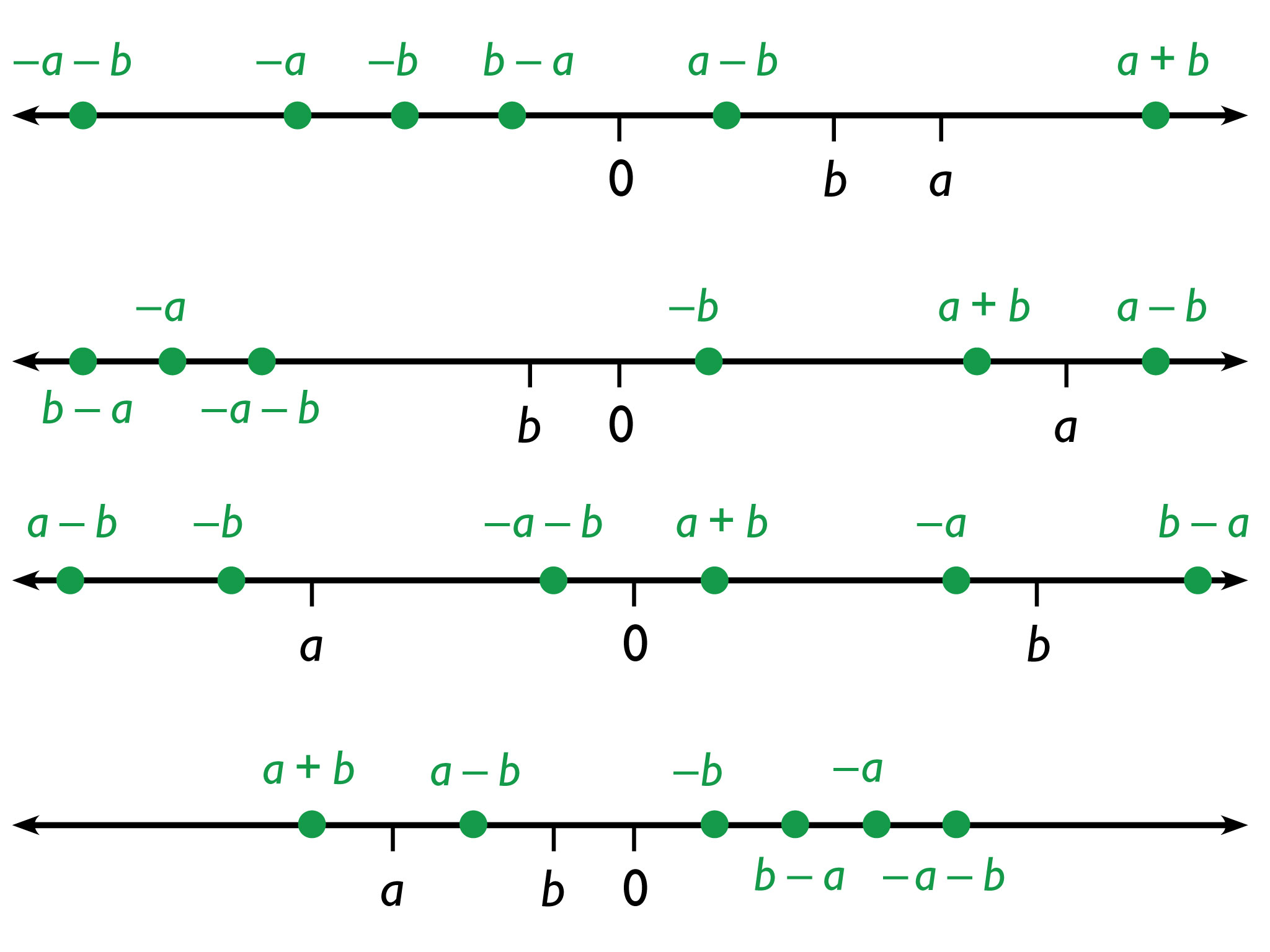2. Opposite expressions:
• aa
• bb
• ab
• a + b
• ab
• ba

# Work Time

1. Four number lines show the position of a and b. On each number line, locate as precisely as you can the following six expressions:
• a
• b
• a – b
• ba
• a + b
• ab
2. Which pairs of expressions are opposites?

HANDOUT: Expressions on a Number Line

# p and n

1. This statement is true for only some values of p and n. Specifically, it is true only when |p| > |n|. Possible explanation: We find p + n by starting at p (which is to the right of 0) and moving left |n| units. When |p| > |n|, we will end up to the right of 0, so the answer is positive. When |p| = |n|, we end up at 0. When |p| < |n|, we end up to the left of 0, so the sum is negative.
2. This is true for all values of p and n. Possible explanation: For any positive value, p, adding n (any negative value) locates the sum to the left of p on the number line since it will move left |n| units. Subtracting n locates the difference to the right of p on the number line since subtracting a negative value is the same as adding that value. Therefore, p + n give a result less than pp.

# p and n

Imagine two numbers, p and n, where p is positive and n is negative.

For the two statements below, decide whether each statement is:

• true for all values of p and n
• true for only some values of p and n
• not true for any values of p and n